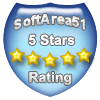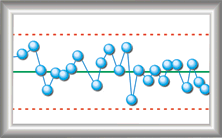# MVPspcShareware - Easy-to-Use SPC Software

## PurposeMVPspc takes the Statistical Process Control Chart and Performance/Capability routines from MVPstats, and puts them in a shareware product. This makes MVPspc great for teaching SPC classes. What makes MVPspc so wonderful? Here are some of the unique features:

• MVPspc uses simple text file input. Data can be copied and pasted directly from a Spreadsheet into MVPspc.
• MVPspc can take data structured in dozens of different formats. For example:
• A variable can be in a single column, where samples can be selected using another variable, a constant sample size, or for use in an individuals chart.
• Sample data can also be entered in a rows, where each row represents a different sample.
• Multiple variables can be in multiple columns, or in a single columns with a grouping variable.
• Control limits may be generated in numerous ways. For example, a Range chart can use the average range, the median range, the average standard deviation, the median standard deviation, the average variance, the average moving range of the ranges, the median moving range of the ranges, the standard deviation of the ranges, or from a know standard deviation. This software is the most flexible to generate control limits -- giving you power that you will not find elsewhere.

## System Requirements

• Windows NT/2000/XP/Vista/Windows 7/Windows 8/Windows 10
• 4.0 MB Hard Disk Space
• Mouse or other pointing device

## Features

### Data Editor

• Create, load, and save data files
• Cut and paste from spreadsheets and other documents
• File length limited only by disk space.
• Assign, read, edit, copy, and paste variable names
• Set missing values
• Conduct analysis using transform equations
• Add transformed columns of data
• Create columns of data from statistics using other columns
• Select data with a "Process If" system

### Control Limit Calculations

• Control Limit Calculations replace the use of tables.
• Numerous methods may be used for each chart. For example the X-bar chart control limits may be generated using the average range, median range average standard deviation, median standard deviation, variance, average moving range of the X-bars, median moving range of the X-bars, and standard deviation of the X-bars.
• The attributes charts have both approximate and exact binomial or Poisson calculations for control limits.
• Estimates of the standard deviation (sigma) are also provided for variables charts.

### Variables Control Charts

• Mean Median, and X (Individuals) charts are available for location
• Range Standard Deviation, Variance, and Moving range charts are available for dispersion
• Options include:
• calculating control limits for the location and dispersion charts independently using a variety of techniques including using the average range average standard deviation, average variance, median range, median standard deviation average or median moving range or standard deviation of the statistic, and user defined values
• connect or not connect points
• display zones
• show all points
• show plus/minus 3 population standard deviation points
• turn control checking on and off
• select the methods used to evaluate control
• generate analysis of the chart including control analysis, capability, location/dispersion correlation, and a oneway random factor analysis of the chart
• generate a grid showing each plotted value, the control limits for each point, and the control assessment
• Add text to individual points

### Attributes Control Charts

• p, np, c and u charts are available
• Options include
• calculating control limits using a variety of techniques including the normal approximation, the exact binomial or Poisson distribution, the median or average moving range or standard deviation of the statistic and user defined values
• connect or not connect points
• display zones
• show all points
• turn control checking on and off
• select the methods used to evaluate control
• Add text to individual points